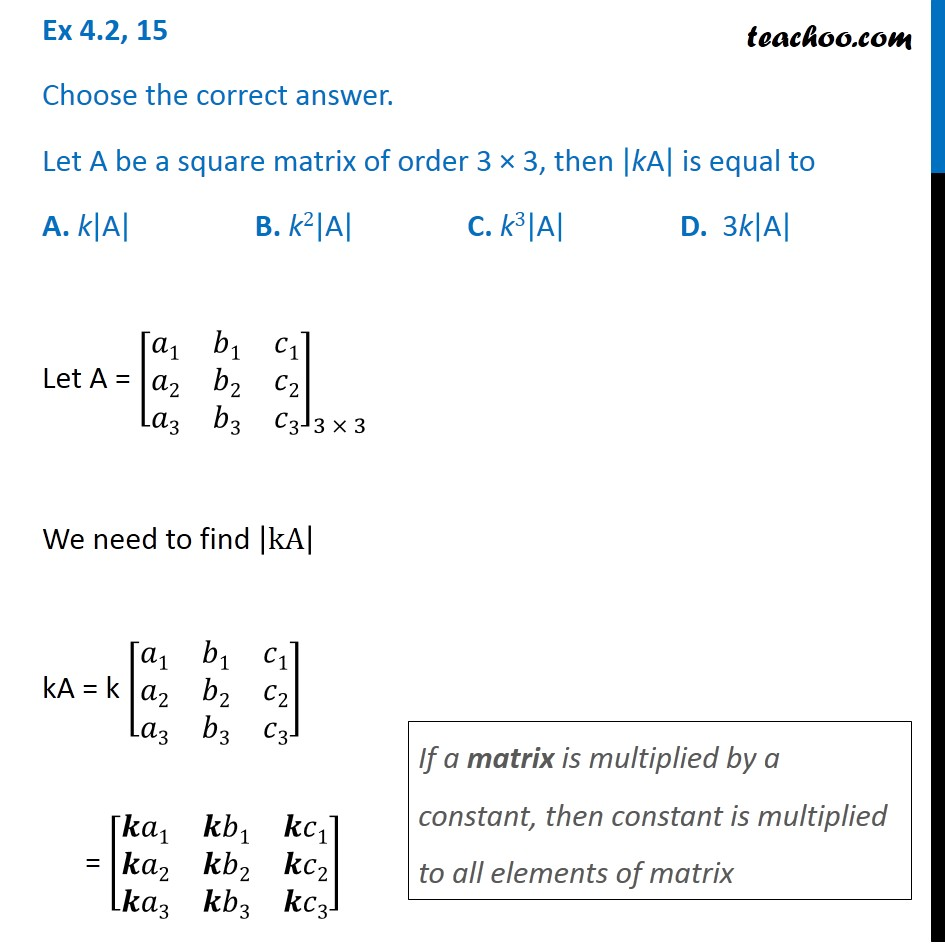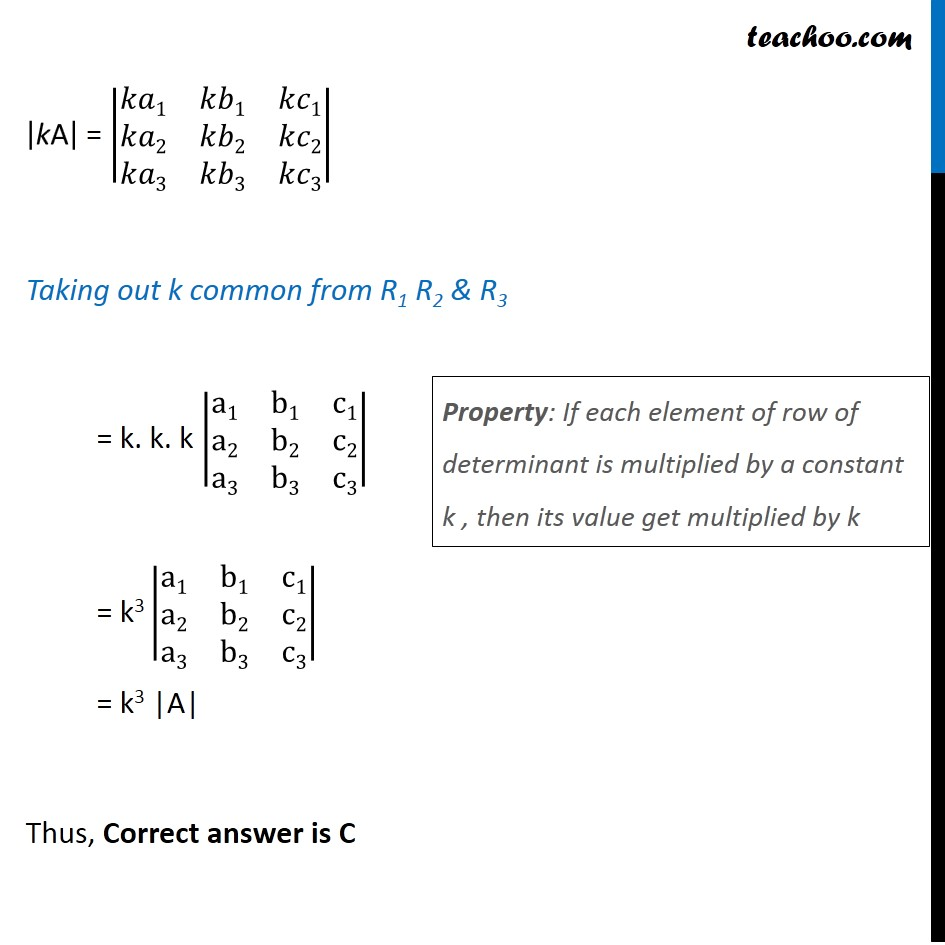Verifying properties of a determinant

Chapter 4 Class 12 Determinants
Concept wiseLearn in your speed, with individual attention - Teachoo Maths 1-on-1 Class

### Transcript

Question 15 Choose the correct answer. Let A be a square matrix of order 3 × 3, then |"kA" | is equal to A. "k" |"A" | B. "k" 2|"A" | C. "k" 3|"A" | D. 3"k" |"A" | Let A = [■8(𝑎1&𝑏1&𝑐1@𝑎2&𝑏2&𝑐2@𝑎3&𝑏3&𝑐3)]_(3 × 3) We need to find |kA| kA = k [■8(𝑎1&𝑏1&𝑐1@𝑎2&𝑏2&𝑐2@𝑎3&𝑏3&𝑐3)] = [■8(𝒌𝑎1&𝒌𝑏1&𝒌𝑐1@𝒌𝑎2&𝒌𝑏2&𝒌𝑐2@𝒌𝑎3&𝒌𝑏3&𝒌𝑐3)] If a matrix is multiplied by a constant, then constant is multiplied to all elements of matrix |"kA" | = |■8(𝑘𝑎1&𝑘𝑏1&𝑘𝑐1@𝑘𝑎2&𝑘𝑏2&𝑘𝑐2@𝑘𝑎3&𝑘𝑏3&𝑘𝑐3)| Taking out k common from R1 R2 & R3 = k. k. k |■8(a1&b1&[email protected]&b2&[email protected]&b3&c3)| = k3 |■8(a1&b1&[email protected]&b2&[email protected]&b3&c3)| = k3 |A| Thus, Correct answer is C Property: If each element of row of determinant is multiplied by a constant k , then its value get multiplied by k